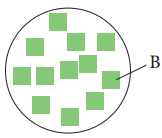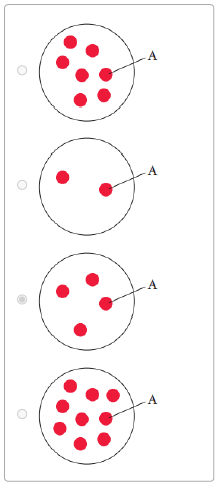# Problem: Consider the generic chemical equation A + 3B → 2C. Let circles represent molecules of A, squares represent molecules of B, and triangles represent molecules of C. The diagram shown below represents the amount of B available for reaction.Select the diagram showing the amount of A necessary to completely react with B.

###### FREE Expert Solution

The given reaction is:

A + 3 B → 2 C

97% (500 ratings)###### Problem Details
Consider the generic chemical equation A + 3B → 2C. Let circles represent molecules of A, squares represent molecules of B, and triangles represent molecules of C. The diagram shown below represents the amount of B available for reaction.Select the diagram showing the amount of A necessary to completely react with B.Frequently Asked Questions

What scientific concept do you need to know in order to solve this problem?

Our tutors have indicated that to solve this problem you will need to apply the Stoichiometry concept. You can view video lessons to learn Stoichiometry. Or if you need more Stoichiometry practice, you can also practice Stoichiometry practice problems.

What professor is this problem relevant for?

Based on our data, we think this problem is relevant for Professor Laviska's class at SHU.